output.to from Sideway
Mechanics: StaticsMechanics Dynamics

12

Draft for Information Only

# Content

```Second Moment of An Area of Geometric Shape  Moment of Inertia of Areas   Second Moment of Area of Rectangle    Second Moment about x' by Double Integration    Second Moment about y' by Single Integration    Second Moment about x by Parallel-Axis Theorem    Second Moment about y by Parallel-Axis Theorem    Polar Moment about C from Rectangular Moments of Inertia    Second Moment of Area of Circle    Second Moment about x'  by Double Integration    Second Moment about y'  by Double Integration    Polar Moment about C from Rectangular Moments of Inertia     Second Moment about A by Parallel-Axis Theorem   Second Moment of Area of Triangle    Second Moment about x' by Single Integration    Second Moment about x by Parallel-Axis Theorem```

# Second Moment of An Area of Geometric Shape

The second moment of an area of a geometric shape can be determined by integration or the parallel-axis theorem. Imply## Moment of Inertia of Areas

### Second Moment of Area of Rectangle

#### Second Moment about x' by Double Integration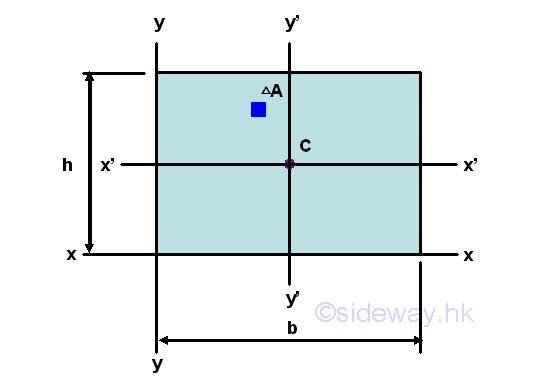The second moment of an area of a rectangle about the centroidal axis x' is#### Second Moment about y' by Single Integration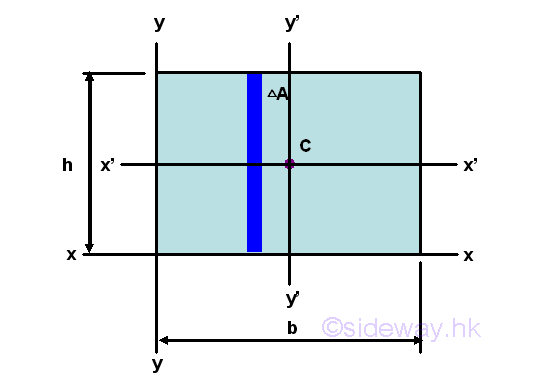The second moment of an area of a rectangle about the centroidal axis y' is#### Second Moment about x by Parallel-Axis Theorem

The second moment of an area of a rectangle about the axis x is#### Second Moment about y by Parallel-Axis Theorem

The second moment of an area of a rectangle about the axis y is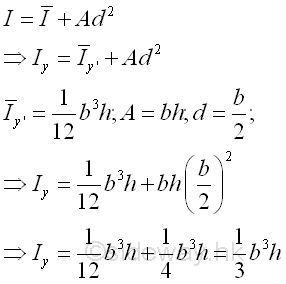#### Polar Moment about C from Rectangular Moments of Inertia

The polar moment of an area of a rectangle about the centroid C is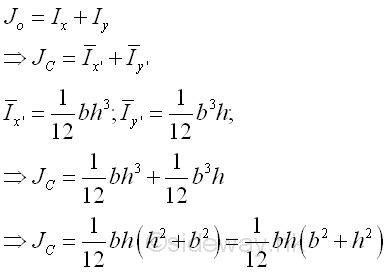### Second Moment of Area of Circle

#### Second Moment about x'  by Double IntegrationThe second moment of an area of a circle about the centroidal axis x' is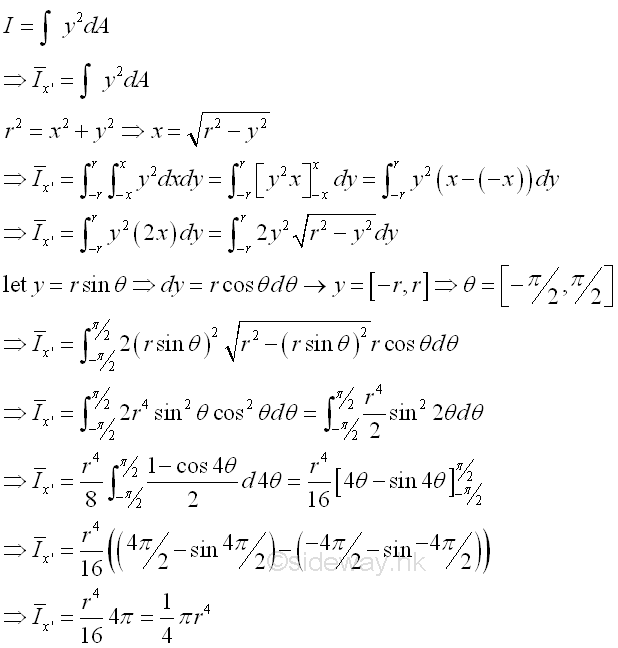#### Second Moment about y'  by Double Integration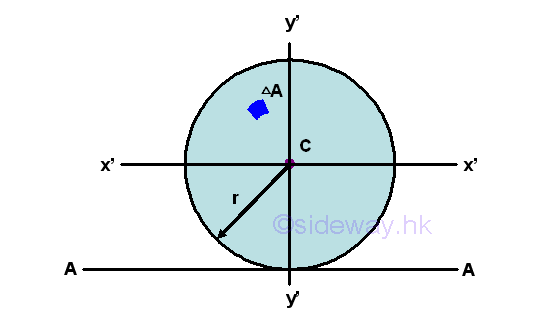The second moment of an area of a circle about the centroidal axis y' is#### Polar Moment about C from Rectangular Moments of Inertia

The polar moment of an area of a circle about the centroid C is#### Second Moment about A by Parallel-Axis Theorem

The second moment of an area of a rectangle about axis A is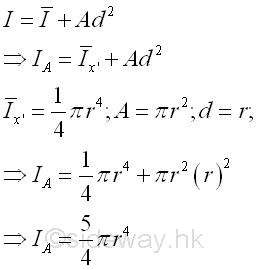### Second Moment of Area of Triangle

#### Second Moment about x' by Single Integration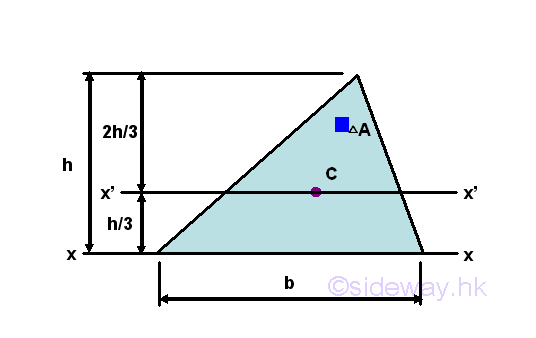The second moment of an area of a triangle about the centroidal axis x' is#### Second Moment about x by Parallel-Axis Theorem

The second moment of an area of a triangle about the axis x is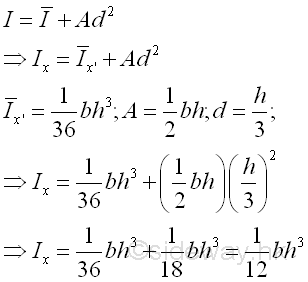ID: 121000007 Last Updated: 10/16/2012 Revision: 0 Ref:References

1. I.C. Jong; B.G. rogers, 1991, Engineering Mechanics: Statics and Dynamics
2. F.P. Beer; E.R. Johnston,Jr.; E.R. Eisenberg, 2004, Vector Mechanics for Engineers: StaticsHome 5

Management

HBR 3

Information

Recreation

Culture

Chinese 1097

English 339

Computer

Hardware 226

Software

Application 213

Latex 52

Manim 205

KB 1

Numeric 19

Programming

Web 289

Unicode 504

HTML 66

CSS 65

SVG 46

ASP.NET 270

OS 429

Python 72

Knowledge

Mathematics

Algebra 84

Geometry 34

Calculus 67

Engineering

Mechanical

Rigid Bodies

Statics 92

Dynamics 37

Control

Natural Sciences

Electric 27